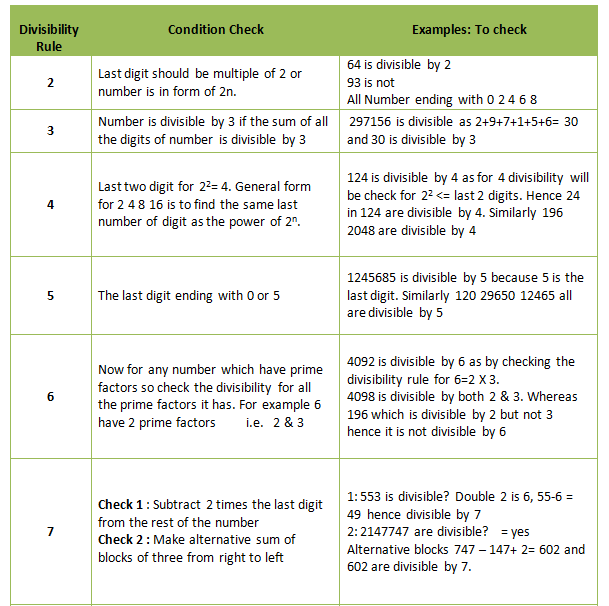Divisibility Rules

A divisibility rule is a short cut for discovering whether a given number is divisible by a fixed divisor without performing the division, usually by examining its digits. Most of the divisibility rules are derived from a concept of remainders.
The two basic rules for finding out whether the number is divisible or not by any particular number:
1.If the divisor is prime number, then we can directly check for it.
2.If the divisor is a composite number then do factorization of divisor into factors, which are co-prime and check the divisibility for each prime factor individually

### Divisibility Rules: 2 to 7### Divisibility Rules: 8 to 12### Divisibility Rules: Special Numbers